• lstm模型代码
千次阅读
2022-02-22 13:56:32

RNN：模型记住所有信息，不管是有用的信息还是无用的信息。

LSTM：引入记忆细胞，具备选择记忆的功能，选择记忆重要信息，过滤噪声信息，减轻记忆负担。

C（t-1）记忆细胞

H（t-1）t-1时刻输出lstm
更多相关内容
• 在时间序列预测问题中，建立LSTM模型，采用python语言代码实现
• 初学者所写，请谨慎尝试，欢迎指出不足！初学者所写，请谨慎尝试，欢迎指出不足！python
• RNN模型与NLP应用笔记(04)：LSTM模型详解与完整代码实现：书接上回，本文开始讲解LSTM的基础内容，同时讲解使用Keras实现LSTM的关键代码以及完整实现。同样是参考李沐大佬和王树森教授的相关课程内容。

# 一、写在前面

书接上回，本文开始讲解LSTM的基础内容，同时讲解使用Keras实现LSTM的关键代码以及完整实现。同样是参考李沐大佬和王树森教授的相关课程内容。

目录

一、写在前面

二、引言

三、LSTM基础知识

四、核心代码详解

五、完整代码实现

六、总结

七、参考文献

# 二、引言

Long short-term memory LSTM是一种RNN模型，是对Simple RNN的改进，LSTM可以避免梯度消失的问题,可以有更长的记忆。

LSTM的论文在1997年发表，也是一种循环神经网络，原理跟simple RNN差不多。如图，每当读取一个新的输入x就会更新状态h，LSTM的结构比Simple RNN要复杂很多，Simple RNN只有一个参数矩阵，LSTM有四个参数矩阵，接下来我们具体来看的LSTM的内部结构。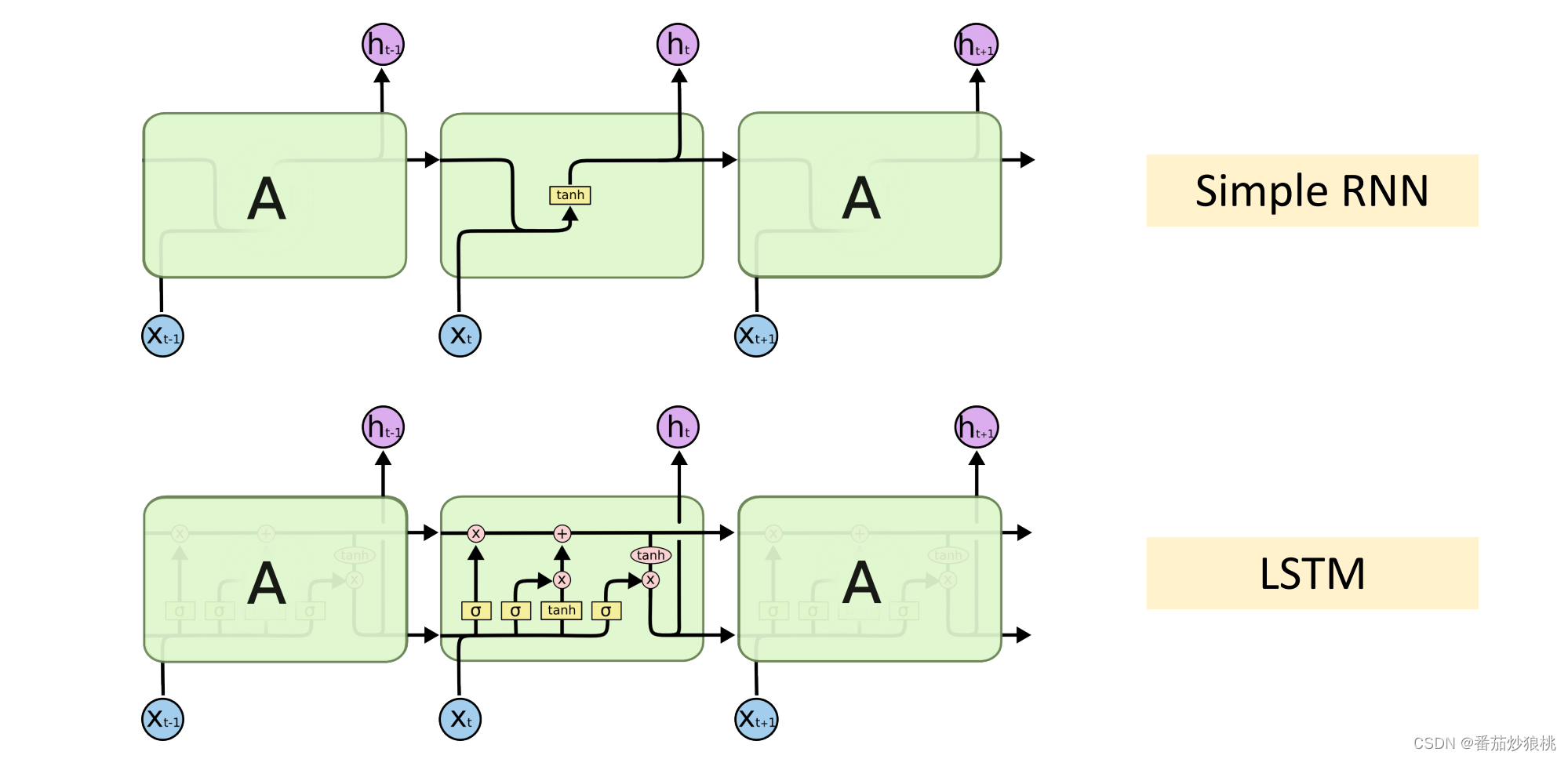# 三、LSTM基础知识

如图，LSTM最重要的设计是这个传输带，记为向量c，过去的信息通过传输带直接送到下一个时刻，不会发生太大的变化，LSTM就是靠传输带来避免梯度消失的问题。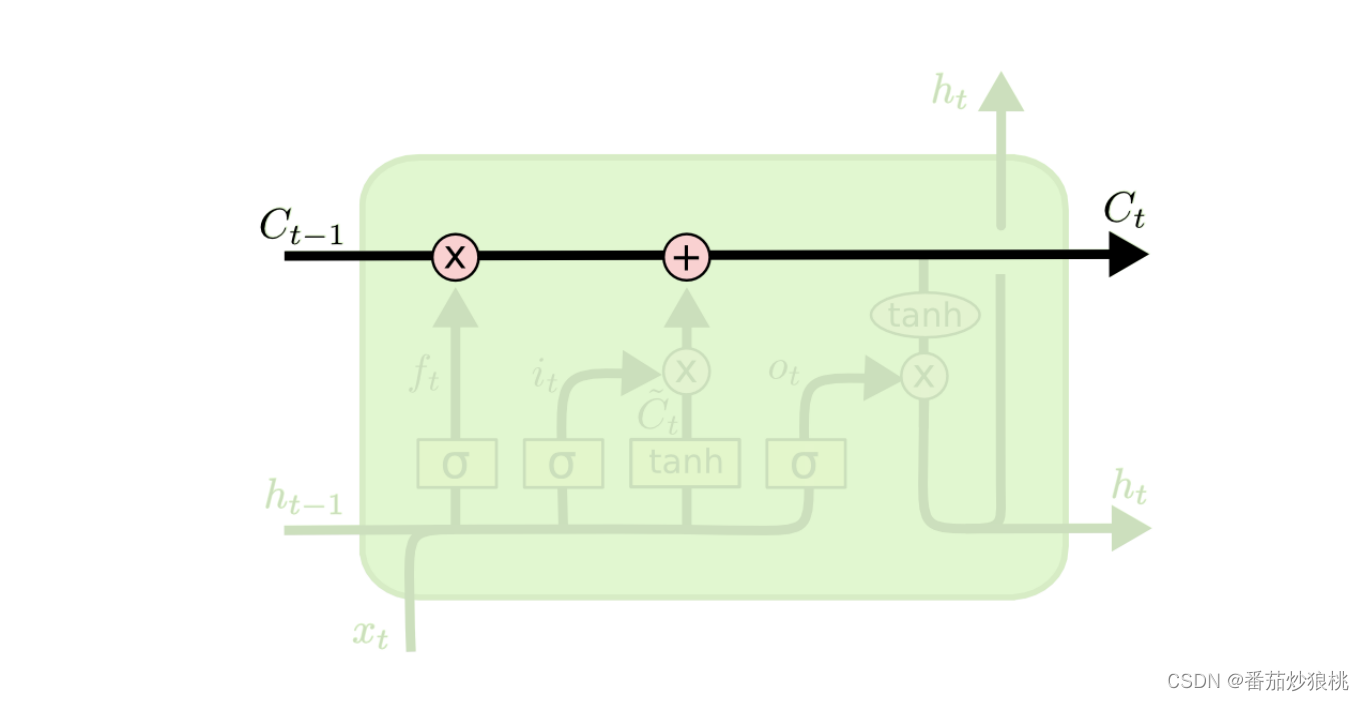如图，LSTM中有很多个Gate，可以有选择的让信息通过，先来看一下forget gate（遗忘门），遗忘门有sigmoid函数和Elementwise multiplication两部分组成。

1Sigmoid函数

输入sigmoid的是一个向量a，sigmoid作用到向量a的每一个元素上，把每一个元素都压到[0,1]之间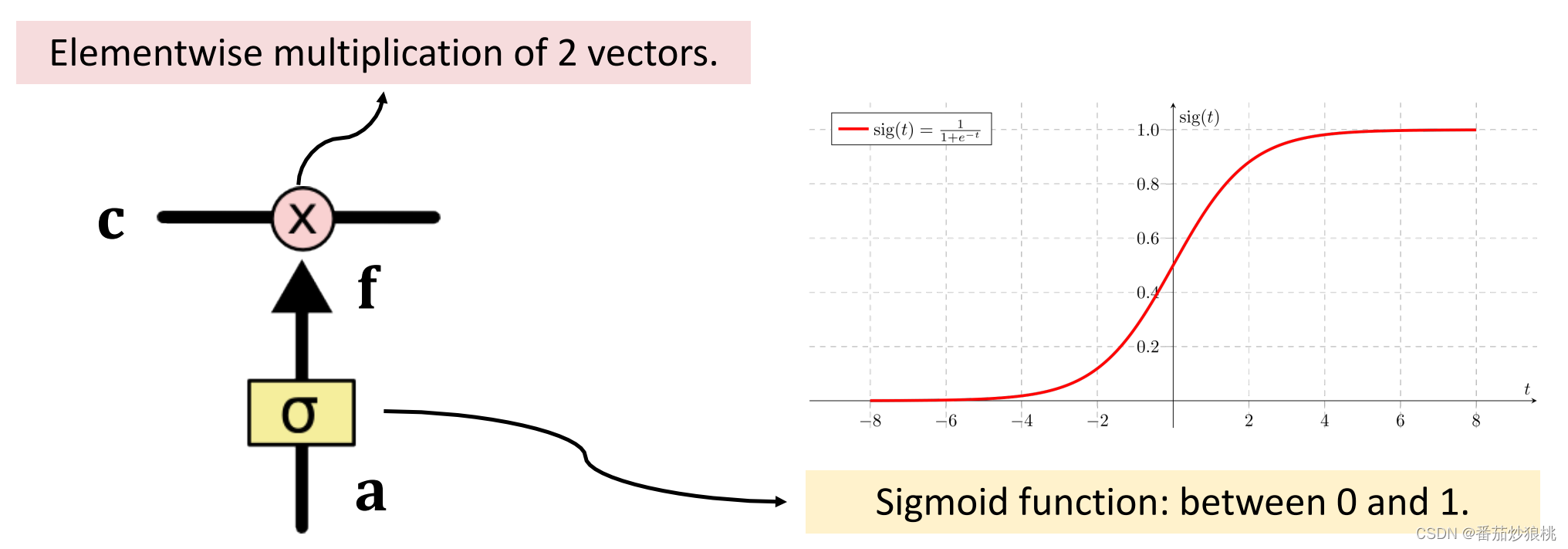举个例子，假如a是下图的向量1，3，0，-2，那么sigmoid函数分别作用在这四个元素上,分别输出0.73、0.95、0.5、0.12这四个数。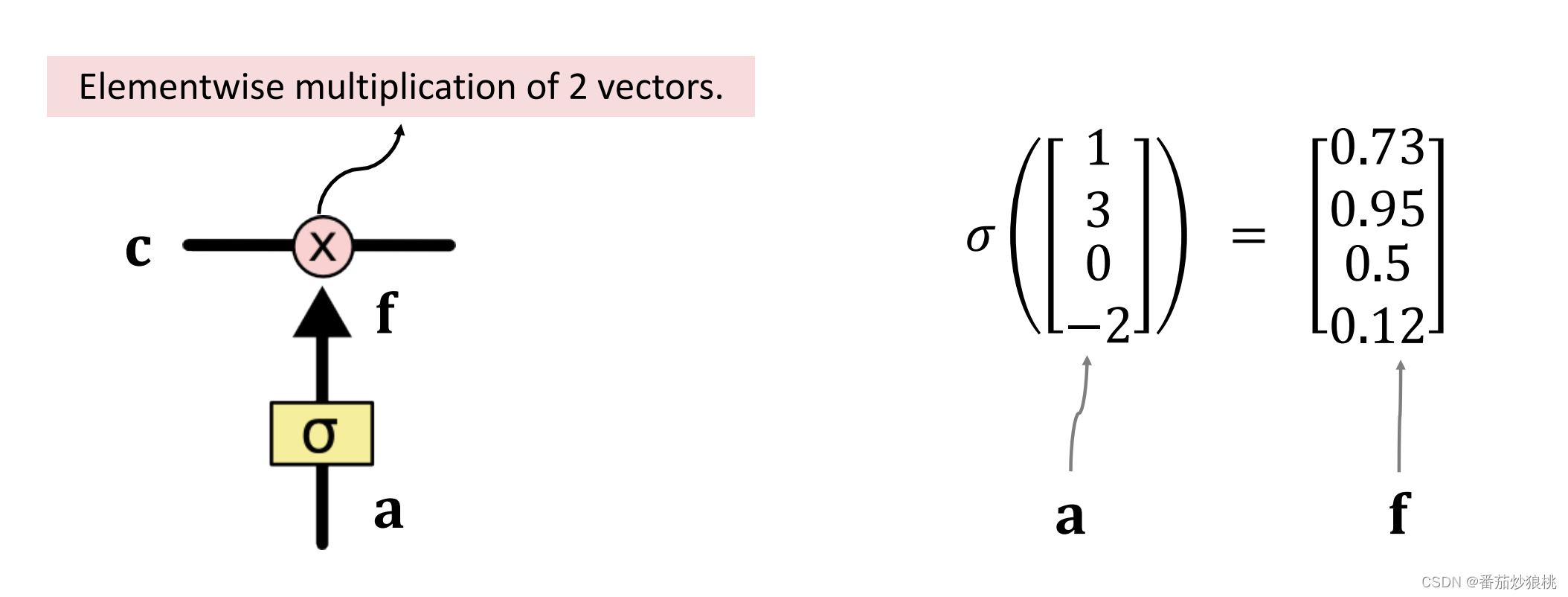输入的向量a与输出的向量f应该有相同的维度。这个例子里，向量a是4维的，向量f也是四维的。

2Elementwise multiplication

算出f向量之后，计算传输带向量c和遗忘门向量f的Elementwise multiplication。

Elementwise multiplication的计算方法如下（举例）：

c和f（有输入a经过sigmoid激活函数得到）都是四维的向量，把它们的每一个元素分别乘起来就行，0.9乘以0.5等于0.45，0.2乘以0等于0，-0.5乘以1等于-0.5以此类推，可以看出Elementwise multiplication的计算结果也是四维的向量。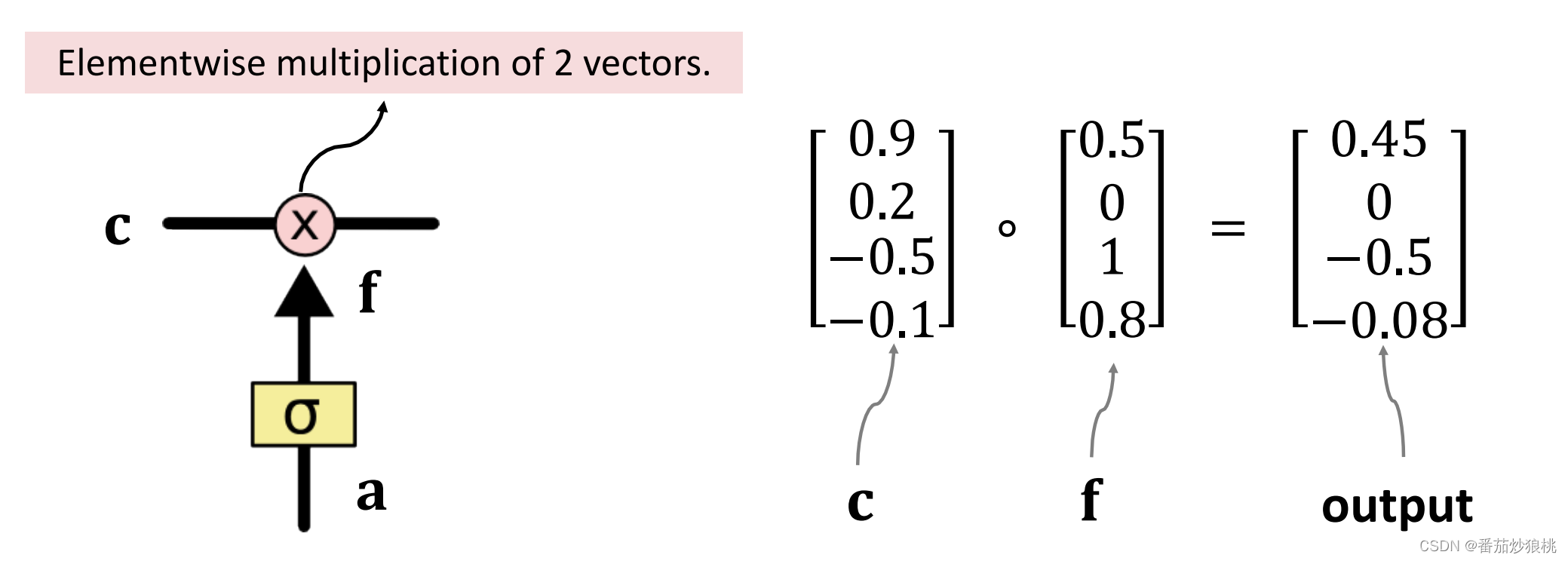遗忘门f，有选择的让传输带c的值通过，假如f的一个元素是0，那么 c对应的元素就不能通过对应的输出是0。假如f的一个元素是1，那么c对应的元素就全部通过，对应的输出是c本身。

3）遗忘门

遗忘门f的计算方法如下，看下面的结构图，ft是上一个状态ht-1与当前输入x的函数，状态ht-1与输入xt做串联操作得到更高维的向量，然后算矩阵Wf与这个向量的乘积，得到一个向量，再用sigmoid的函数得到向量ft。ft的每一个元素都介于0和1之间。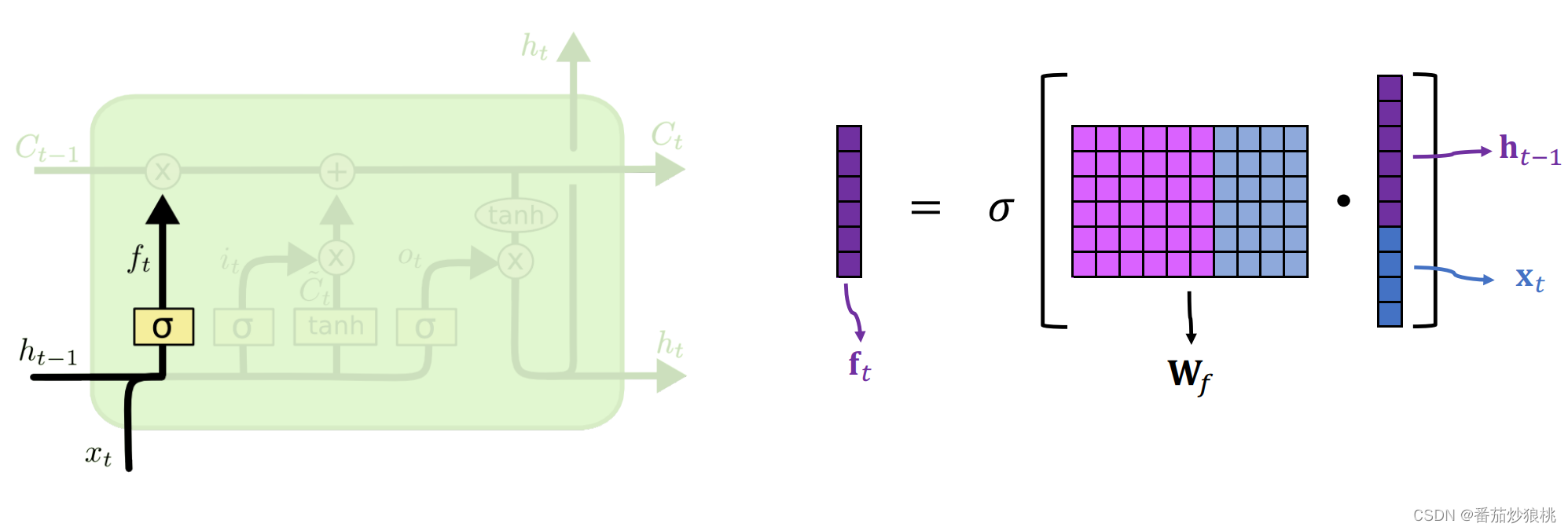遗忘门有一个参数矩阵Wf, 需要通过反向传播从训练数据里学习

刚才讲了遗忘门，现在来看一看input gate输入门

4）输入门

下面这张结构处理输入门 it依赖于旧的状态向量ht-1和新的输入xt，输入门it的计算很类似遗忘门，把旧的状态ht-1与新的输入xt做串联，得到更高为的向量 然后算矩阵Wi与这个向量的乘积得到一个向量，最后再用sigmoid函数得到向量it，it的每一个元素都介于0和1之间。

输入门也有自己的参数矩阵，记为wiwi也需要从训练数据中学习，还需要算new value它是个向量计算，跟遗忘门和输入门都很像，也是把旧状态ht-1，与新输入xt做串联操作，再乘以参数矩阵。区别在于激活函数不是Sigmoid，而是双区正切函数tanh，所以算出的向量的元素都介于[-1,1]之间，同时如图可知计算该向量ct’也需要单独的一个参数矩阵，记作wc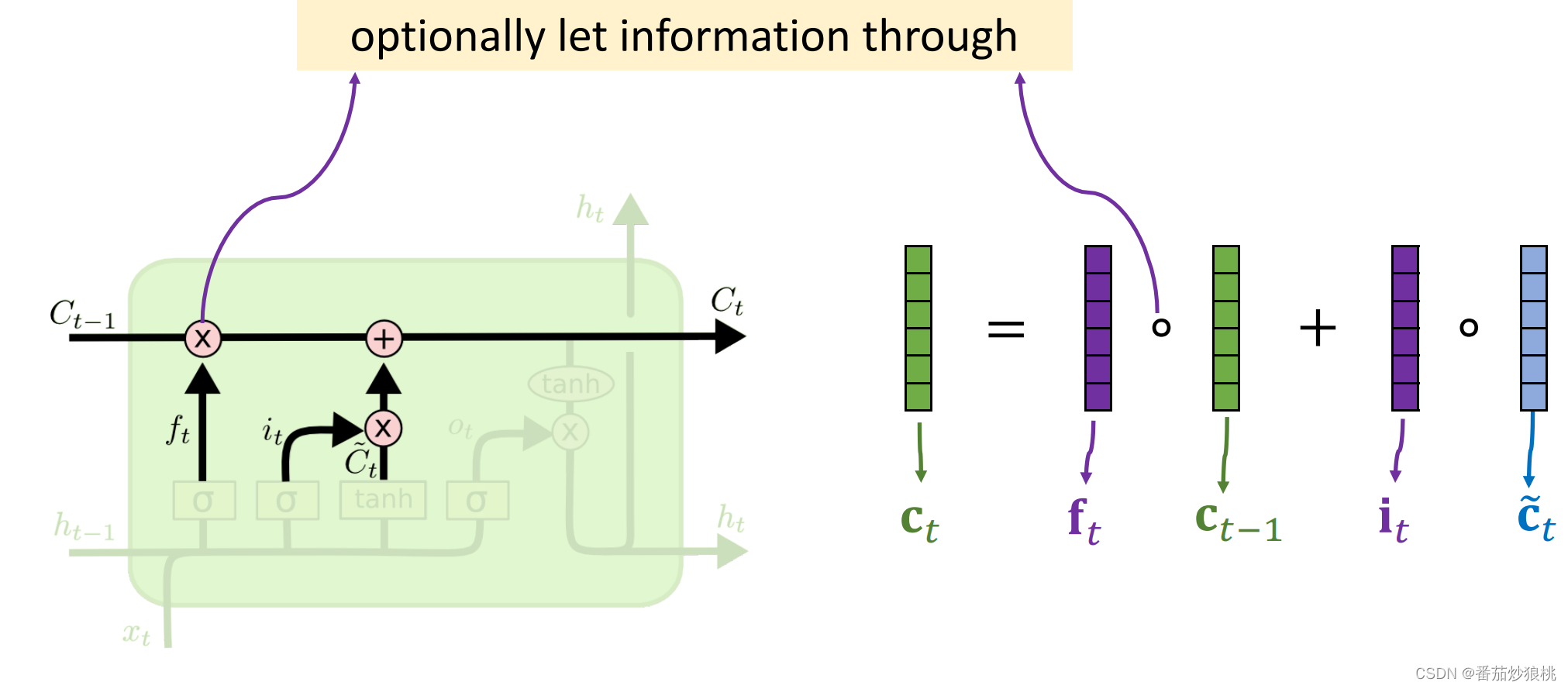在介绍了上面的步骤以后，如上图，我们已经可以知道遗忘门ft，输入门it，以及New value：ct’，还知道传输带旧的值ct-1，现在可以更新传输带c了。

5ct的计算

传输带ct的更新如下：用遗忘门ft和传送带旧的值ct-1算Elementwise multiplication。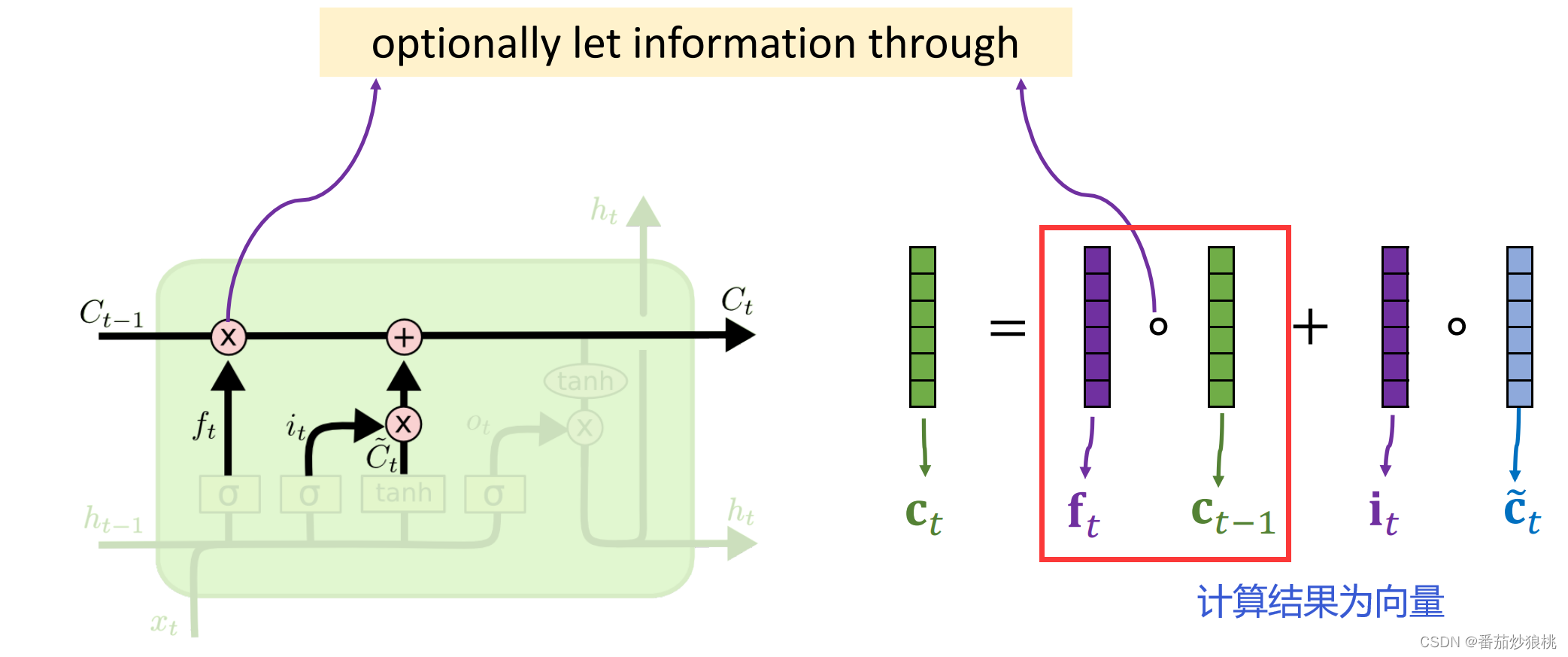遗忘门ft和传送带ct-1是维度相同的向量，算出的成绩也是个向量，遗忘门ft 可以选择性的遗忘ct-1中的一些元素

如果ft中的一个元素是0那么ct-1相应的元素就会被遗忘，刚才选择性遗忘掉了传数带ct-1的一些元素，现在要往传输带上添加新的信息。

计算输入门it和新的值ct’的Elementwise multiplication、输入门it和新的值ct’，都是维度相同的向量，它们的乘积也是维度相同的向量，把乘积加到传送带上就行了。这样就完成了对传送带的一轮更新，用遗忘门删除了传送带上的一些信息，然后加入新的信息，得到了传输带新的值ct’，现在已经更新完传送带c了。

最后一步是计算LSTM的输出，也就是状态向量ht，ht是这么计算的呢？

5LSTM的输出ht的计算

首先计算outpuit gate输出门的向量Ot，输出门的向量Ot跟前面的遗忘门、输入门的计算基本一样, 把旧的状态ht-1与新的输入st做串联，得到更高为的向量，然后算矩阵wo与这个向量的乘积得到一个向量，最后再用sigmoid函数得到向量Ot。Ot的每一个元素都介于0和1之间。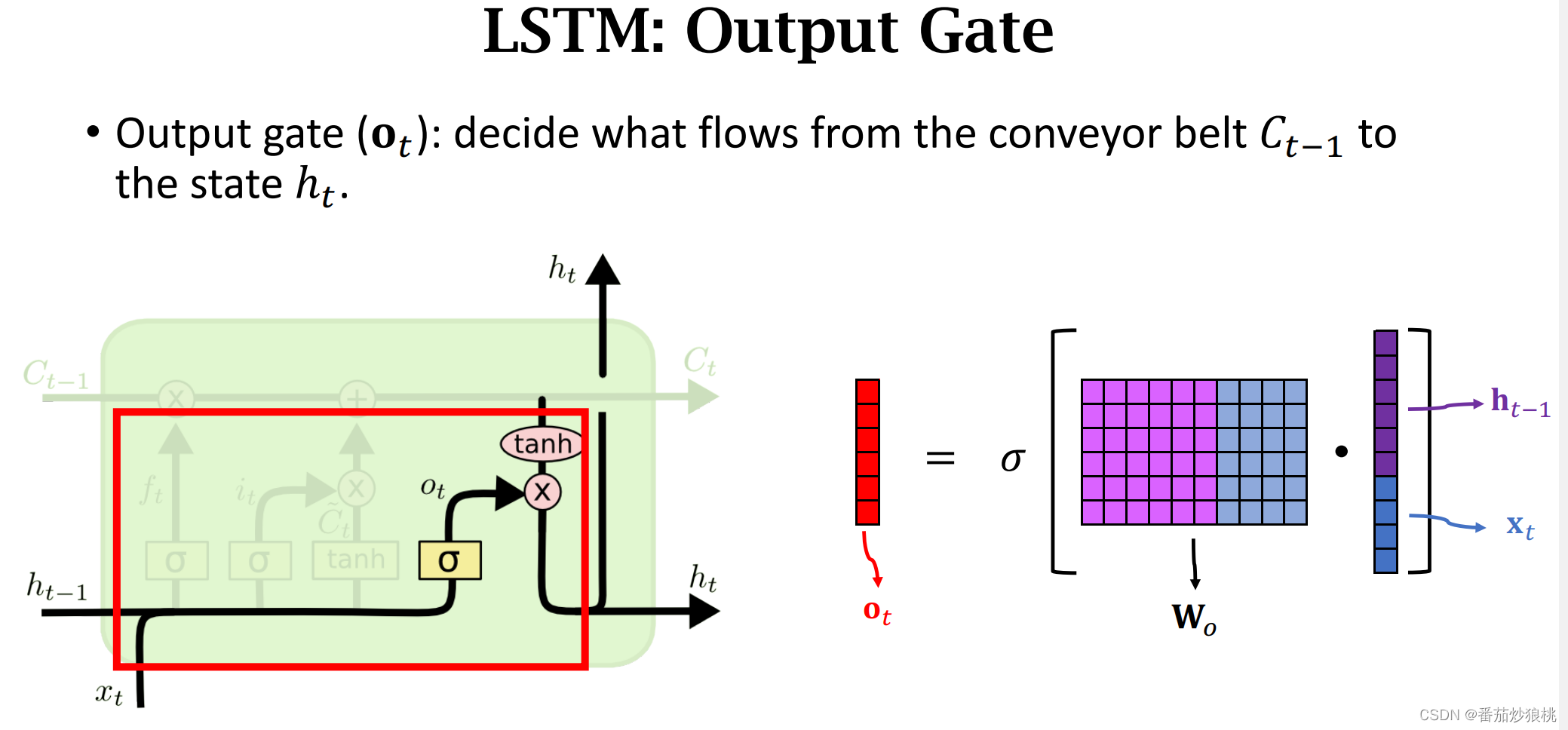输出门也有自己的参数矩阵wo，也需要从训练数据中学习

如下图，现在计算状态向量ht，对传输带ct的每一个元素求双区正切函数，把元素全都压到[-1,1]的区间，然后求这两个向量的Elementwise multiplication，下如的红色向量是刚刚求出的输出门ot，这样就得到了状态向量ht

看一下结构图ht它有两份copies，一份copy传输到了下一步，另一份copy成了LSTM的输出。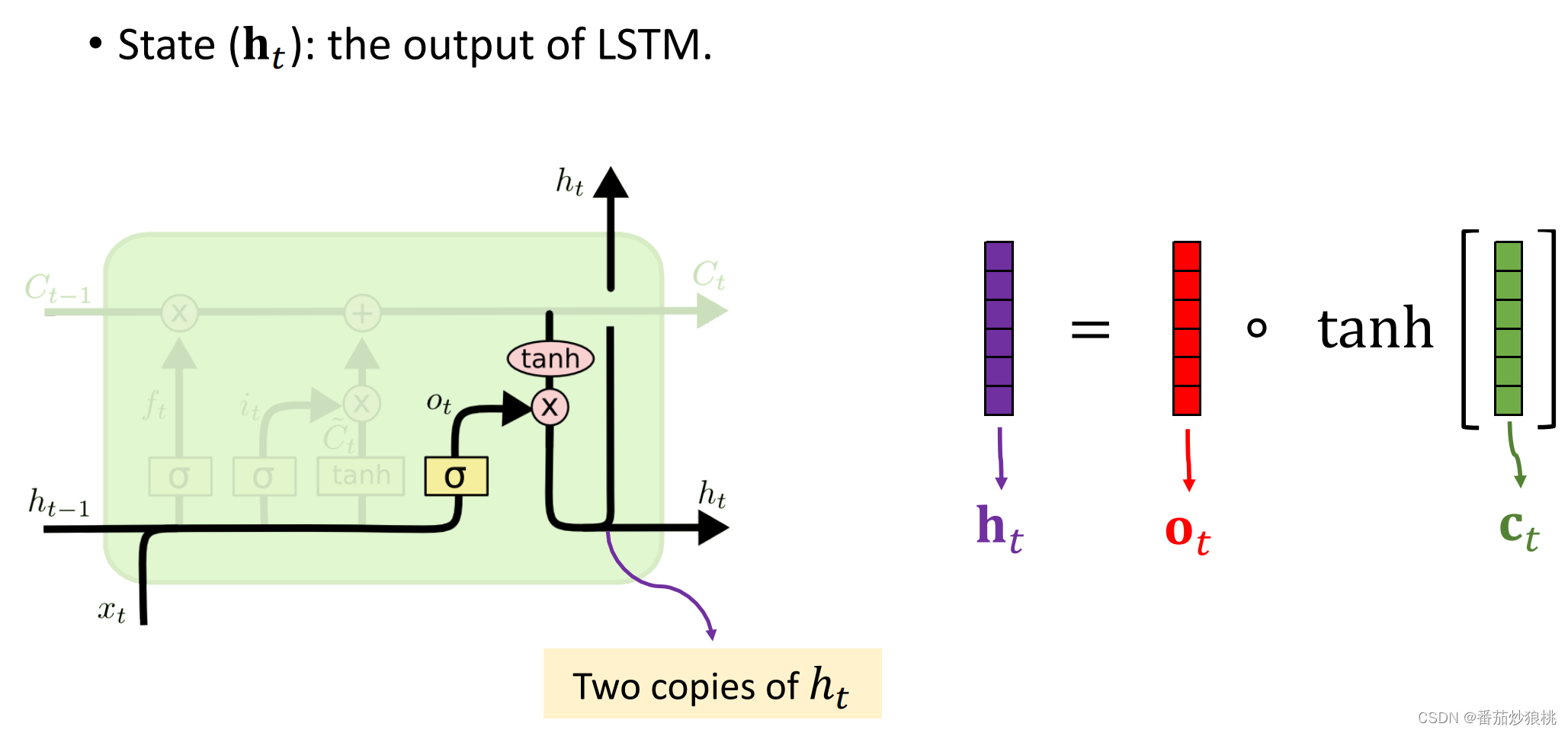可见目前位置，一共有7个向量 x输入了LSTM，可以认为，所有这些x向量的信息都积累在了状态ht里面。

我们来算一下LSTM的参数数量

LSTM有遗忘门、输入门、 new value以及输出门，这四个模块都有各自的参数矩阵w，所以一共有四个参数矩阵。矩阵的行数是h的维度，列数是h的维度+上x的维度，所以l s tm参数的数量是4×h的维度×（h的维度+x的维度）。

# 四、核心代码详解

利用Keras实现，代码关键步骤详解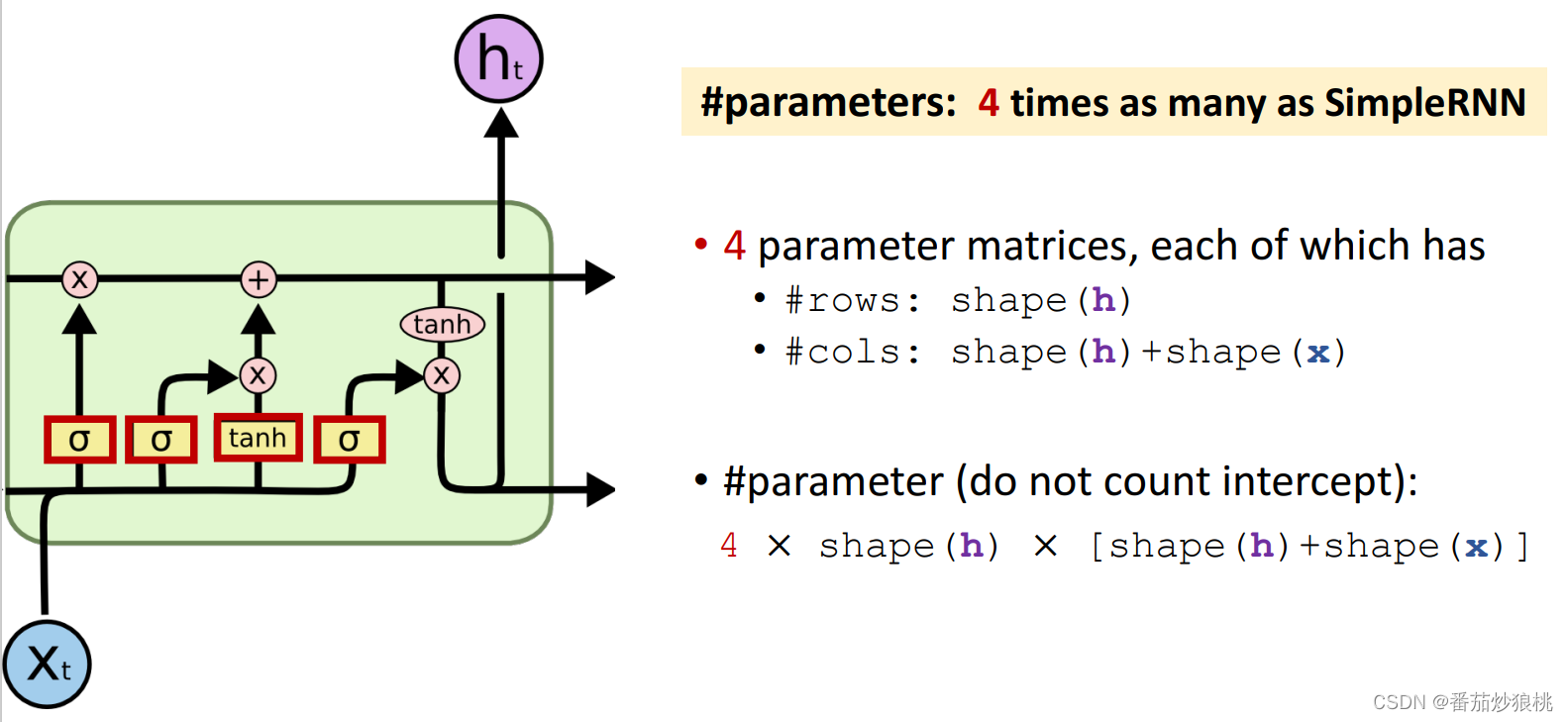虽然LSTM的结构非常复杂，但是用Keras实现起来非常简单,跟simple RNN的实现几乎完全一样。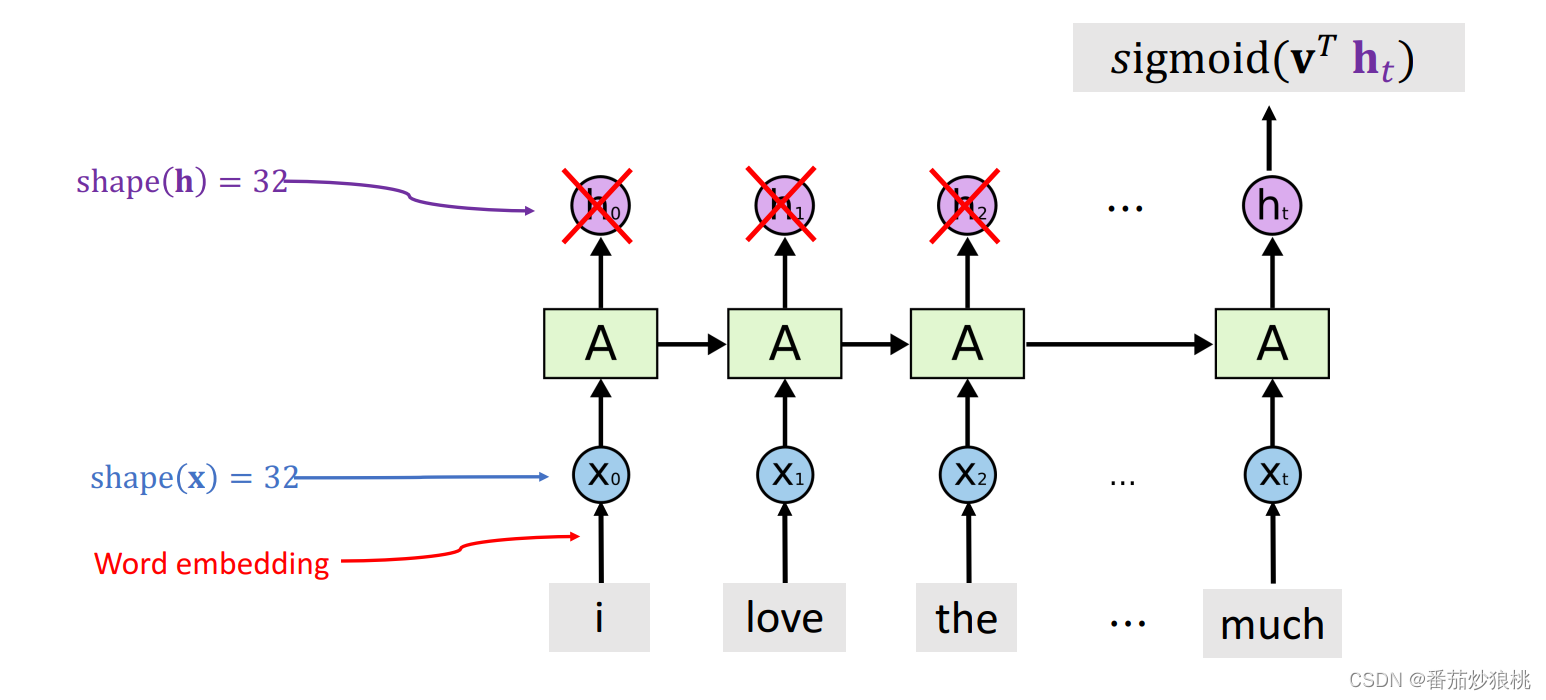拿LSTM来判断电影评论是正面还是负面，跟用sim RNN一样，让LSTM只输出最后一个状态向量ht，ht就是从一段500个词的电影评论中提取的特征向量，然后输入线性分类器来判断评论是正面的还是负面的。

Kearas代码是这样的, 跟用simple RNN完全一样，只是把这个层的名字换成了LSTM而已, 其余地方全都一样。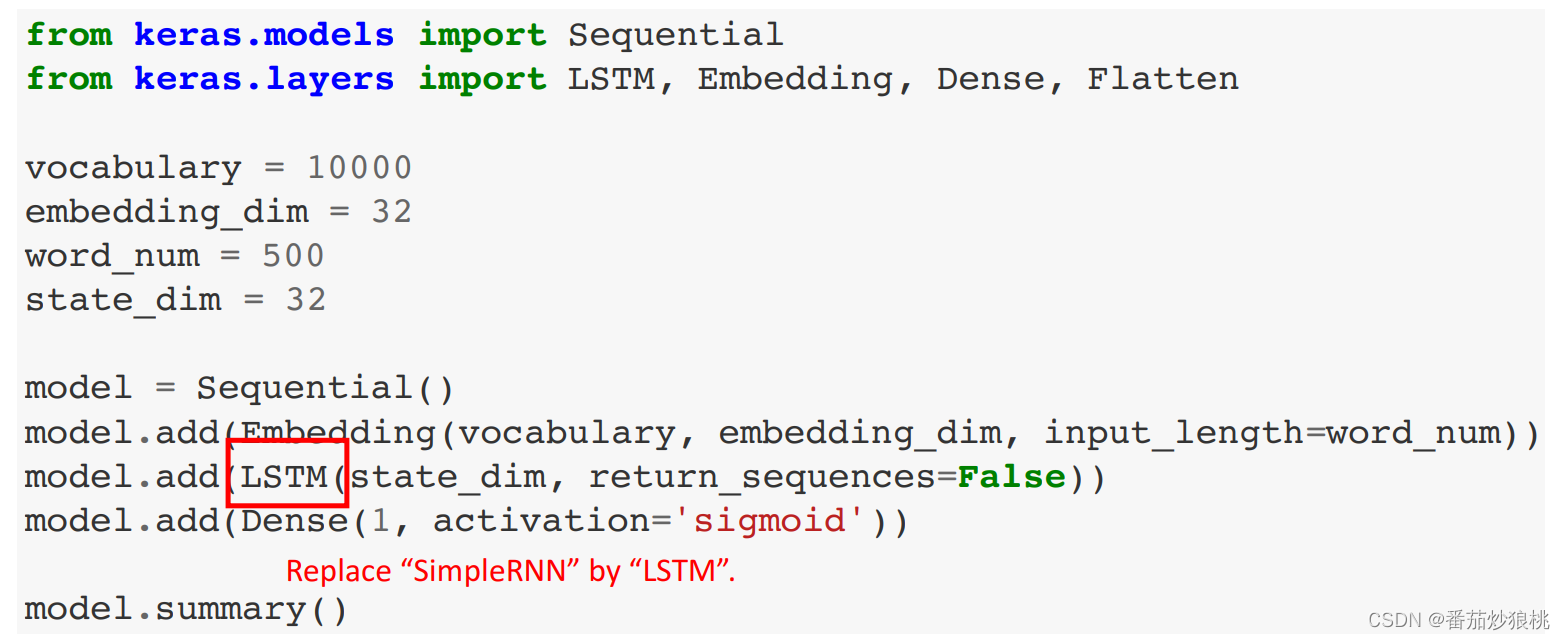下图神经网络的结构，这是LSTM层，让LSTM只输出最后一个状态向量h，所以这一层的输出是一个32维的向量。

来算一下模型参数，每个参数矩阵是h的维度32乘以h的维度32+ x的维度32。Keras默认使用偏移量，所以参数里还有个32维的向量，一个矩阵和一个向量加起来总共有2080个参数，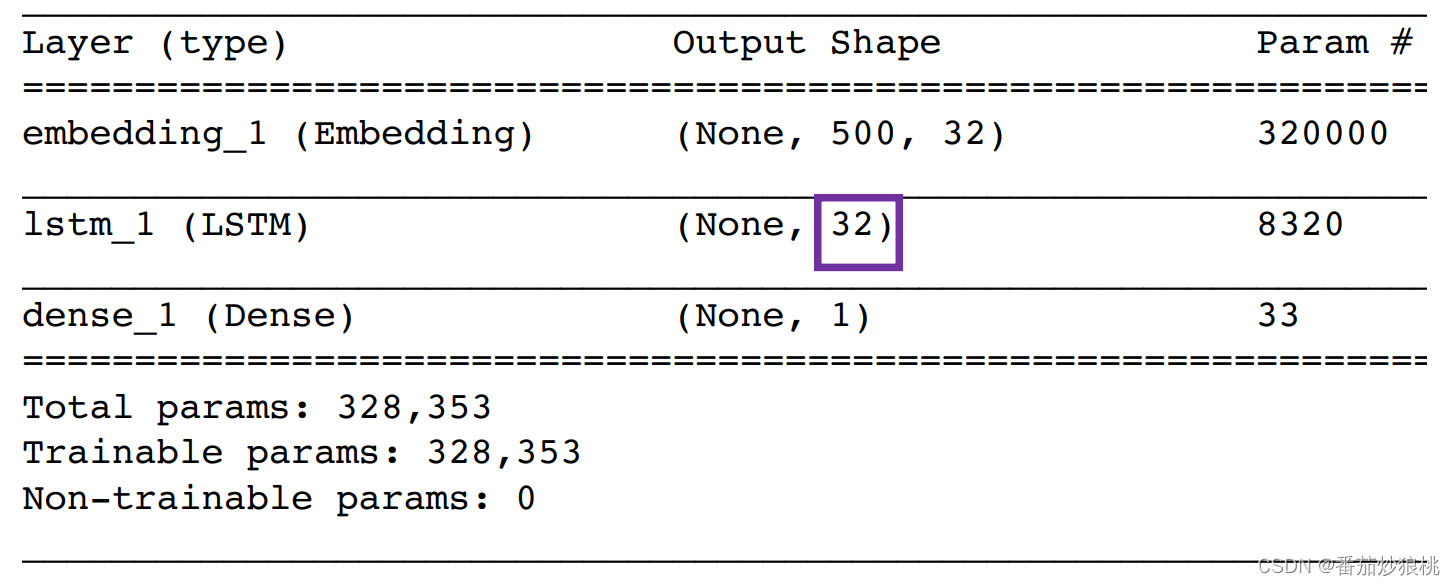LSTM一共有四个参数矩阵和四个Intersect向量，所以参数的数量等于2080*4=8320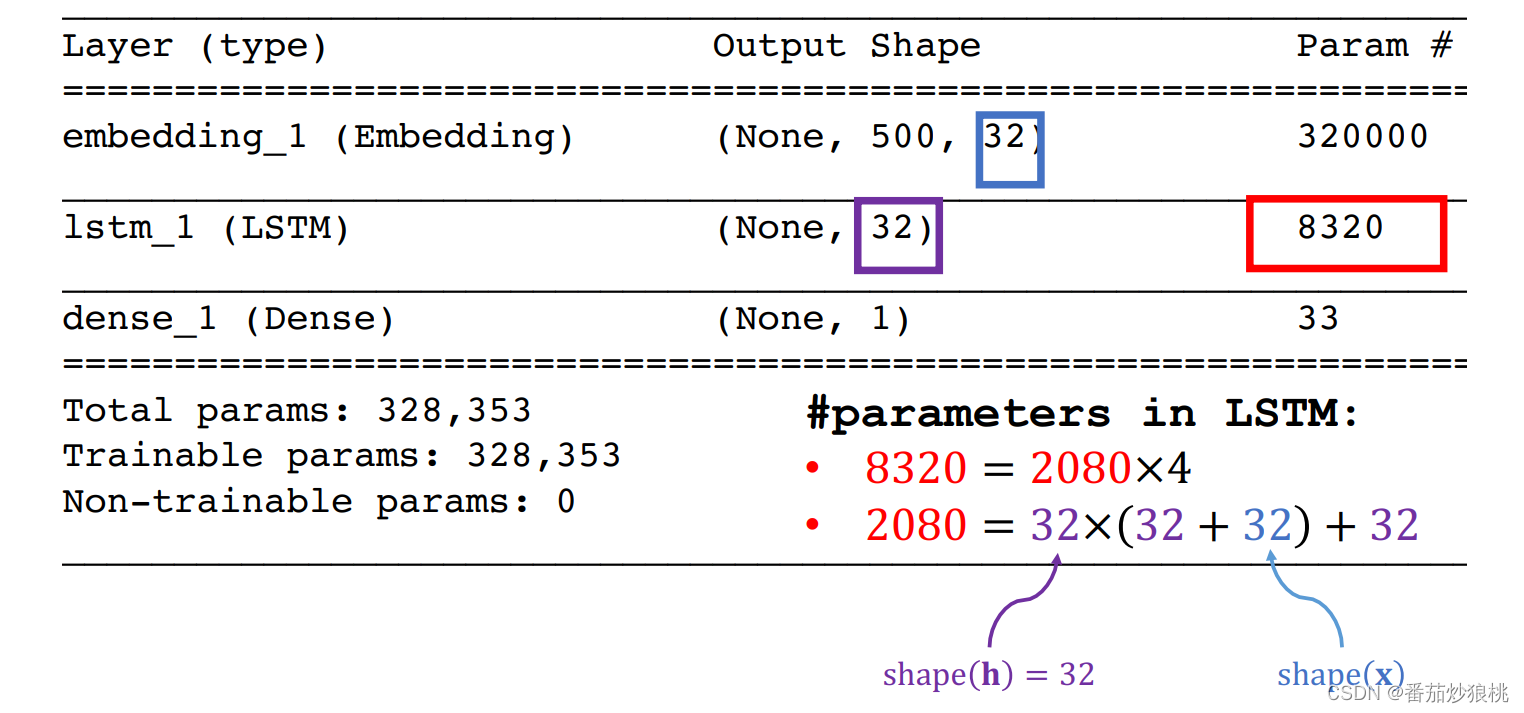下图是在IMDB电影评论数据上的结果，训练准确率是91.8%,validation和测试的准确率都是88.6%左右，这比simple RNN有些提升，simple RNN的测试准确率是84%。

Dropout也可以用在LSTM层上，具体方法比较复杂，但实现很简单，如下图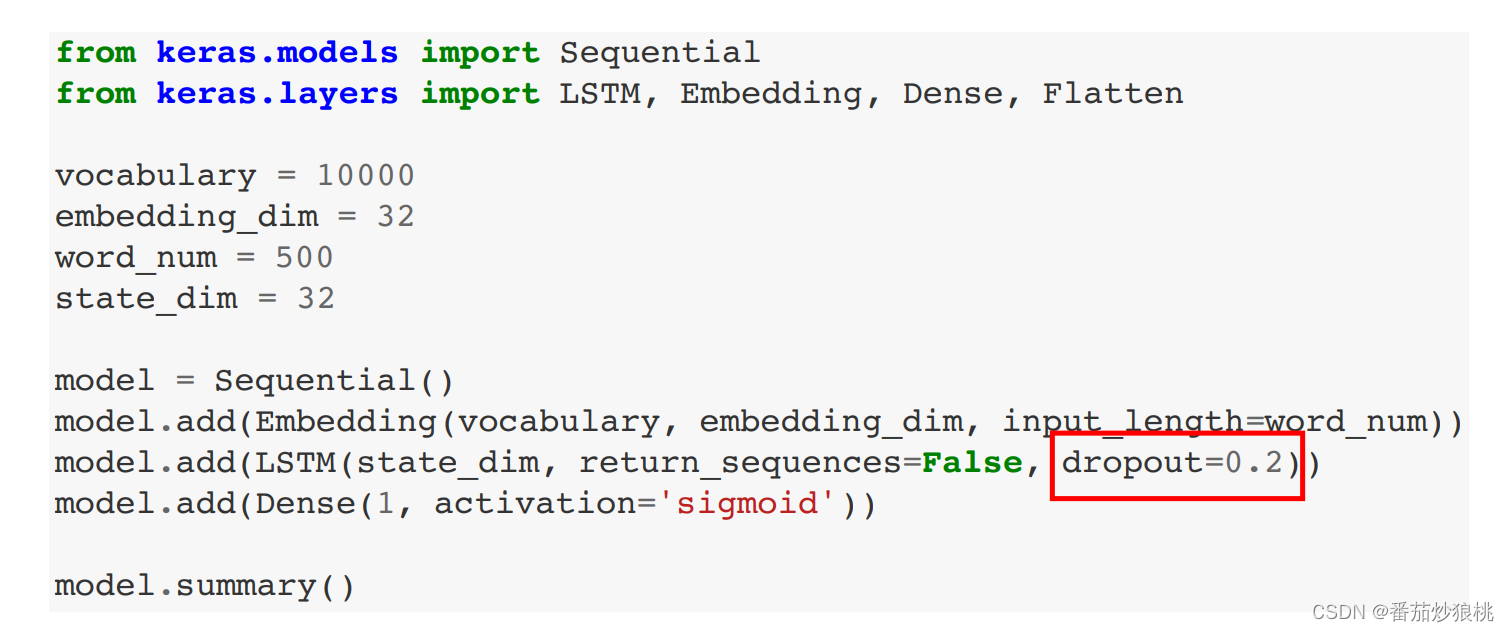只需要加上Dropout等于某个0-1之间的数字就行了，然而做实验的时候发现使用Dropout并没有提升测试准确率，原因是这样的：虽然训练的时候出现了过拟合，但是过拟合不是由LSTM层造成的，而是由Embedding造成的。

LSTM有8000多个参数，而引白领层却有32万个参数，在这里对LSTM使用Dropout没有帮助的，因为过拟合不是由LSTM层造成的。

# 五、完整代码实现

此处完整代码实现部分的数据处理内容和上篇内容一致，此处直接给出。

代码大部分内容和Simple RNN相同，仅需稍加改变模型定义模块

'数据预处理模块'
'数据集读取与预处理'
# 此处使用Keras库自带函数进行简洁实现(从零开始实现请看上一节)
# 使用keras的embedding层处理文字数据（同样使用imdb数据集）

from keras.datasets import imdb
from keras import preprocessing

max_feature = 10000  # 词汇量（作为特征的单词个数）
maxlen = 500  # 在500个单词以后截断文本

(input_train, y_train), (input_test, y_test) = imdb.load_data(num_words=max_feature)
# y_train、y_test分别表示训练集和测试集的标签
# max_words=10000：只考虑数据集中前10000个最常见的单词
print(len(input_train), 'train sequences')
print(len(input_test), 'test sequences')

print('sequence 格式：（samples*time）')
input_train = preprocessing.sequence.pad_sequences(input_train, maxlen=maxlen)
input_test = preprocessing.sequence.pad_sequences(input_test, maxlen=maxlen)
# 此处相当于对齐序列（补0或者阶段评论）
print('input_train shape:', input_train.shape)
print('input_test shape:', input_test.shape)

'LSTM层的应用'
from keras.layers import Dense, LSTM, Embedding
from keras.models import Sequential

model = Sequential()

model.compile(optimizer='rmsprop', loss='binary_crossentropy', metrics=['accuracy'])
history = model.fit(
input_train, y_train,
epochs = 10,
batch_size = 128,
validation_split = 0.2
)
model.summary()
'查看模型最终性能'
loss_and_acc = model.evaluate(input_test, y_test)
print('loss=' + str(loss_and_acc))
print('acc=' + str(loss_and_acc))


代码运行结果如下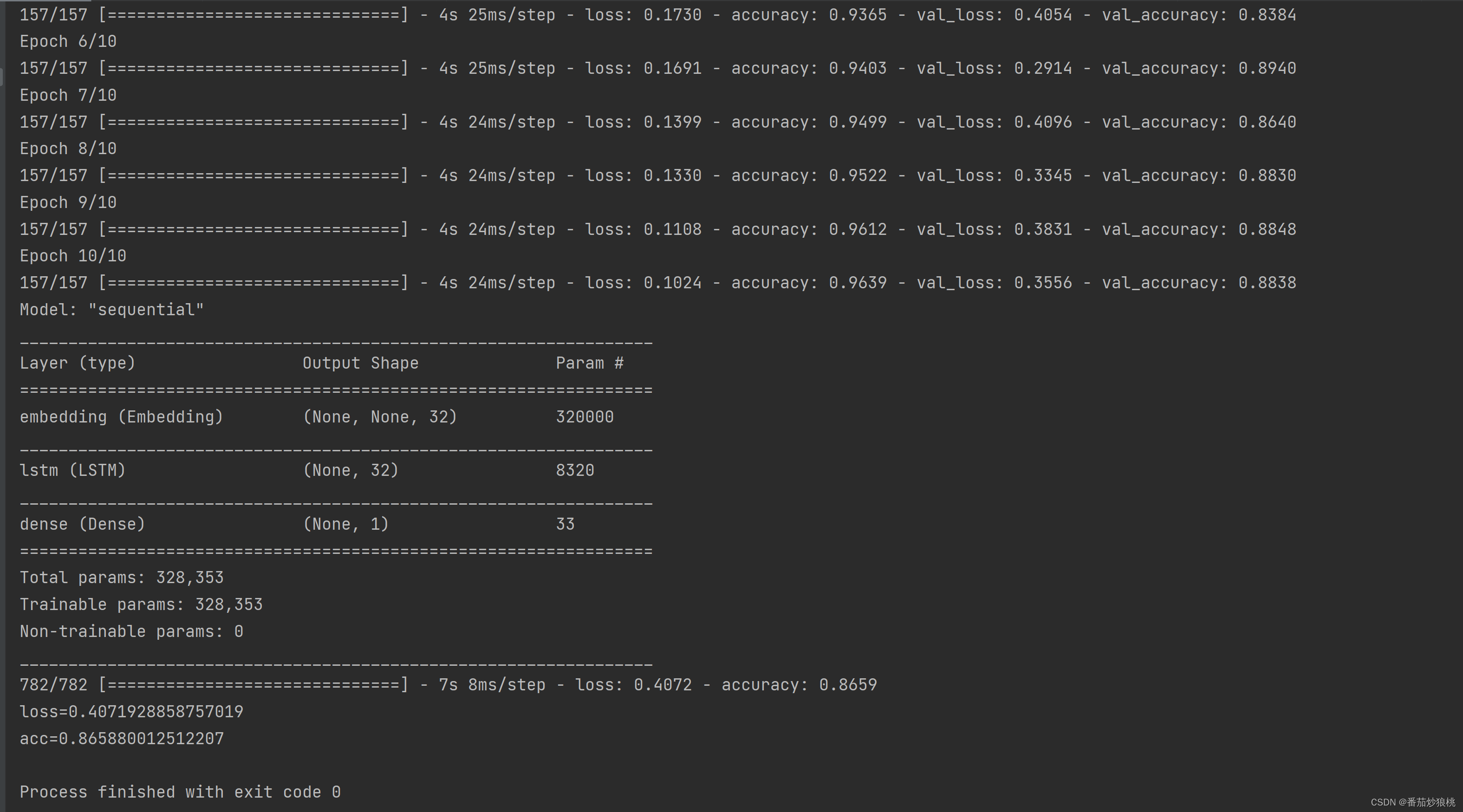# 六、总结

总结一下本文内容，本文讲了LSTM模型和用Keras的实现

（1）LSTM和simpleRNN主要的区别是用了一条传输带，让过去的信息可以很容易传输到下一时刻，这样就有了更长的记忆。LSTM的表现总是比simple RNN要好，所以想用RNN的时候，就用LSTM模型，而不要用Simple RNN模型。

（2）LSTM有四个组件，分别是遗忘门，输入门，new value新的输入，以及输出门，这四个组建各自有一个参数矩阵，所以一共有四个参数矩阵。

（3）LSTM参数的数量是4×h的维度×（h的维度+x的维度），

4 × shape(h) × [shape(h)+shape(x)]

下一篇内容讲Bidirectional RNN

# 七、参考文献

Tensorflow+Keras入门练习（六）：文字处理（嵌入层，RNN，LSTM） - 知乎

GitHub - wangshusen/DeepLearning

展开全文lstm 自然语言处理 神经网络
• 长短时记忆网络（ Long short-term memory，LSTM ）是一种循环神经网络 (Recurrent neural network, RNN)的特殊变体。

目录

模型原理

模型实现

导入所需要的库

设置随机数种子

导入数据集

打印前五行数据进行查看

数据处理

归一化处理

查看归一化处理后的数据

将时间序列转换为监督学习问题

打印数据前五行

划分训练集和测试集

查看划分后的数据维度

搭建LSTM模型

得到损失图

模型预测

画图展示

得到预测图像

回归评价指标

# 模型原理

长短时记忆网络（ Long short-term memory，LSTM ）是一种循环神经网络 (Recurrent neural network, RNN)的特殊变体，具有“门”结构，通过门单元的逻辑控制决定数据是否更新或是选择丢弃，克服了 RNN 权重影响过大、容易产生梯度消失和爆炸的缺点，使网络可以更好、更快地收敛，能够有效提高预测精度。LSTM 拥有三个门， 分别为遗忘门、输入门、输出门，以此决定每一时刻信息记忆与遗忘。输入门决定有多少新的信息加入到细胞当中，遗忘门控制每一时刻信息是否会被遗忘，输出门决定每一时刻是否有信息输出。其基本结构如图所示。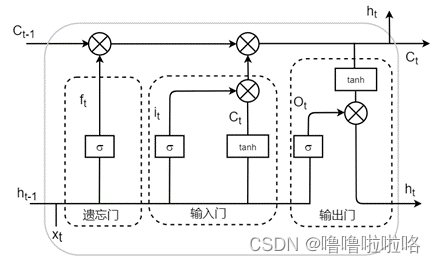公式如下：

（1）遗忘门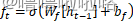（2）输入门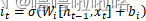（3）单元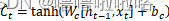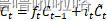（4）输出门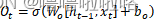（5）最终输出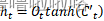# 模型实现

## 导入所需要的库

import matplotlib.pyplot as plt
from pandas import read_csv
from pandas import DataFrame
from pandas import concat
from sklearn.preprocessing import MinMaxScaler
from tensorflow.keras.models import Sequential
from tensorflow.keras.layers import LSTM,Dense,Dropout
from numpy import concatenate
from sklearn.metrics import mean_squared_error,mean_absolute_error,r2_score
from math import sqrt


## 设置随机数种子

import tensorflow as tf
tf.random.set_seed(2)

## 导入数据集

qy_data=read_csv(r'C:\Users\HUAWEI\Desktop\abc.csv',parse_dates=['num'],index_col='num')
qy_data.index.name='num' #选定索引列

### 打印前五行数据进行查看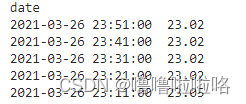## 数据处理

# 获取DataFrame中的数据，形式为数组array形式
values = qy_data.values
# 确保所有数据为float类型
values = values.astype('float32')

## 归一化处理

使用MinMaxScaler缩放器，将全部数据都缩放到[0,1]之间，加快收敛。

scaler = MinMaxScaler(feature_range=(0, 1))
scaled = scaler.fit_transform(values)

### 查看归一化处理后的数据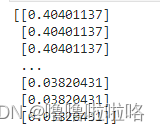## 将时间序列转换为监督学习问题

时间序列形式的数据转换为监督学习集的形式，例如：[,,,,]转换为[[0,10],[10,11],[11,12],[12,13],[13,14]]，即把前一个数作为输入，后一个数作为对应输出。

def series_to_supervised(data, n_in=1, n_out=1, dropnan=True):
n_vars = 1 if type(data) is list else data.shape
df = DataFrame(data)
cols, names = list(), list()
# input sequence (t-n, ... t-1)
for i in range(n_in, 0, -1):
cols.append(df.shift(i))
names += [('var%d(t-%d)' % (j + 1, i)) for j in range(n_vars)]
# forecast sequence (t, t+1, ... t+n)
for i in range(0, n_out):
cols.append(df.shift(-i))
if i == 0:
names += [('var%d(t)' % (j + 1)) for j in range(n_vars)]
else:
names += [('var%d(t+%d)' % (j + 1, i)) for j in range(n_vars)]
# put it all together
agg = concat(cols, axis=1)
agg.columns = names
# drop rows with NaN values
if dropnan:
agg.dropna(inplace=True)
return agg

reframed = series_to_supervised(scaled, 2, 1)

### 打印数据前五行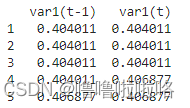## 划分训练集和测试集

# 划分训练集和测试集
values = reframed.values
trainNum = int(len(values) * 0.7)
train = values[:trainNum,:]
test = values[trainNum:, :]

### 查看划分后的数据维度

print(train_X.shape, train_y.shape)
print(test_X.shape, test_y.shape)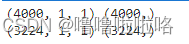## 搭建LSTM模型

初始化LSTM模型，设置神经元核心的个数，迭代次数，优化器等等

model = Sequential()
history = model.fit(train_X, train_y, epochs=95, batch_size=2, validation_data=(test_X, test_y), verbose=2,shuffle=False)

### 得到损失图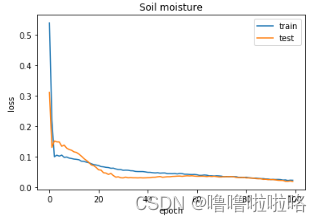​​​​​​​

## 模型预测

y_predict = model.predict(test_X)
test_X = test_X.reshape((test_X.shape, test_X.shape))

## 画图展示

plt.figure(figsize=(10,8),dpi=150)
plt.plot(inv_y,color='red',label='Original')
plt.plot(inv_y_predict,color='green',label='Predict')
plt.xlabel('the number of test data')
plt.ylabel('Soil moisture')
plt.legend()
plt.show()

### 得到预测图像

将测试集的y值和预测值绘制在同一张图表中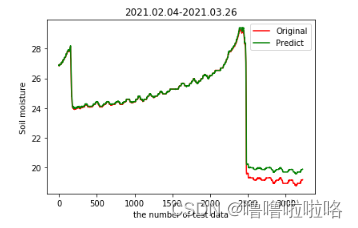## 回归评价指标

# calculate MSE 均方误差
mse=mean_squared_error(inv_y,inv_y_predict)
# calculate RMSE 均方根误差
rmse = sqrt(mean_squared_error(inv_y, inv_y_predict))
#calculate MAE 平均绝对误差
mae=mean_absolute_error(inv_y,inv_y_predict)
#calculate R square
r_square=r2_score(inv_y,inv_y_predict)
print('均方误差MSE: %.6f' % mse)
print('均方根误差RMSE: %.6f' % rmse)
print('平均绝对误差MAE: %.6f' % mae)
print('R_square: %.6f' % r_square)

展开全文lstm
• ## Attention-LSTM模型的python实现

千次阅读 热门讨论 2022-05-27 13:19:54
Attention-LSTM模型分为输入层、LSTM 层、Attention层、全连接层、输出层五层。LSTM 层的作用是实现高层次特征学习；Attention 层的作用是突出关键信息；全连接层的作用是进行局部特征整合，实现最终的预测。 这里...

# 1.模型结构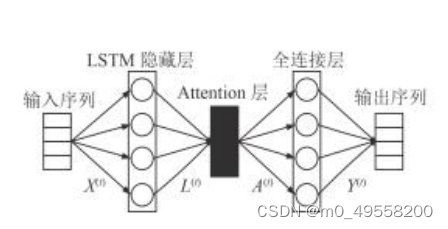Attention-LSTM模型分为输入层、LSTM 层、 Attention层、全连接层、输出层五层。LSTM 层的作用是实现高层次特征学习； Attention 层的作用是突出关键信息；全连接层的作用是进行局部特征整合，实现最终的预测。
这里解决的问题是：使用Attention-LSTM模型进行数据的预测。完整的代码在文末展示

# 1.输入层

输入层是全部特征进行归一化之后的序列。归一化的目的是保证特征处于相似的尺度上，有利于加快梯度下降算法运行速度。可以使用MAX-MIN归一化的方法。归一化用EXCEL公式即可做到。

# 2.LSTM层

LSTM 单元内部引入了门的机制来做到信息的保护和控制，包括遗忘门、输入门和输出门。其中，输入门用于控制记忆单元更新的信息量；遗忘门用于控制前一时刻记忆单元信息被保留的量；输出门用于控制输出至下一隐藏状态的信息量；Cell 则通过控制不同的门实现信息的存储与删除。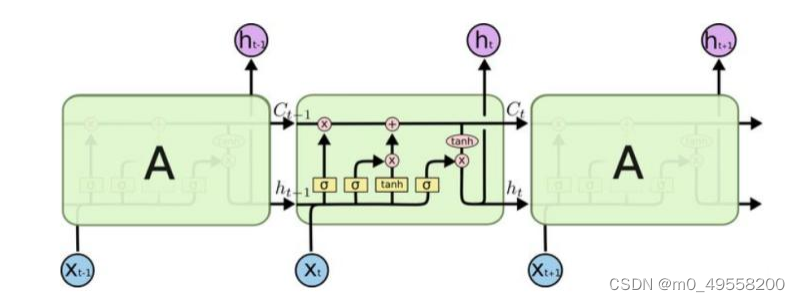这里直接使用了keras包中自带的LSTM函数。LSTM层的代码如下所示：

inputs = tf.constant(x, dtype=tf.float32, shape=[1, 30025, 16])
# print("输入：", inputs.shape)
# print("输入：", inputs)
lstm = tf.keras.layers.LSTM(4, return_sequences=True)
output = lstm(inputs)
# print("LSTM特征提取结果：", output)#LSTM特征提取结果
###############################LSTM

这里解释一下几个参数。tf.constant函数中shape=[1，30025，16]。1表示数据的批数，30025为输入数据的条数，16为每条数据的特征数。tf.keras.layers.LSTM函数的第一个参数4表示输出向量的维数为4

# 3.Attention层

将LSTM 层的输出向量做为 Attention 层的输入。注意力机制的本质为计算某一特征向量的的加 权求和。 本文采用的是乘法注意力机制中的 Scaled Dot-Product Attention 方法，其计算主要分为 3 个步骤：
1. 将 query 和每个 key 进行点积计算得到权重
2. 使用 Softmax 函数对权重归一化处理。
3. 将权重和对应的 value 加权求和获得 Attention
综上所述，Attention 层输出的计算公式如下：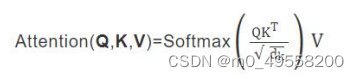其中，Q 为查询向量序列、 k 为键向量序列、 V 为值向量序列、 dk Q K V 向量的维度。 Q、 K、 V 的计算方法如下所示：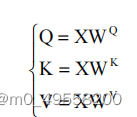输入数据 WQWKWV 和矩阵相乘得到 QKV。实际上就是输入数据经过线性变换得到 Q，K，VX 为输入的数据。因此Attention层的输出向量维度也是4。设计 Attention 层时最重要的是设计权重矩阵 WQWKWV。他们与输入数据决定了 QKV的向量。不同的权重分配对预测有不同的效果。

Attention层的python实现如下：

def softmax(x):
x_exp = np.exp(x)
# 如果是列向量，则axis=0
x_sum = np.sum(x_exp, axis=0, keepdims=True)
s = x_exp / x_sum
return s

wq = np.array([[0.2, 0.2, 1, 0], [0.3, 0.2, 0.3, 1], [1, 0, 1, 0.1], [0.1, 0, 1, 1]])
wk = np.array([[0.3, 0, 1, 0], [0.3, 1, 0, 1], [1, 0, 1, 0], [1, 0.1, 0, 1]])
wv = np.array([[1, 0, 0.5, 0], [0, 1, 0.2, 1], [1, 0, 1, 0], [0.1, 1, 0.1, 0]])  # 权重矩阵
x = output.numpy()
q = np.dot(x, wq)
# print(q)
k = np.dot(x, wk)
v = np.dot(x, wv)

a = 0
attention = []

while (a < 30025):
temp1 = np.dot(q[a], k[a].T)
temp2 = softmax(temp1) / 2
temp3 = np.dot(temp2, v[a])
attention.append(temp3)
a = a + 1
# print("attention输出",attention)#attention层输出
#################################Attention

此处wq，wk，wv三个矩阵需要自行调试。softmax函数的目的是将多分类的结果以概率的形式展现出来，是Attention层公式中的一个步骤。

计算输出结果attention矩阵时是将三万余条数据一条一条计算的，而没有将所有LSTM层的输出数据其看成一个大矩阵放入attention层进行计算。因为这样计算的复杂度太大，会报错，无法计算。

# 4.全连接层

将注意力机制层的输出结果做为全连接层的输入，并将每次输入对应的归一化之后的对地速
度引入，训练模型。故全连接层有 4 个输入。全连接层的输出，也就是整套模型的输出为一个元素，即归一化后的预测 值。将其反归一化后即可得出预测结果。
def re(x):
s = 15.1 * x + 1.6
return s

input_nodes = 4
hidden_nodes = 3
output_nodes = 1

learning_rate = 0.005

n = test.neuralNetwork(input_nodes, hidden_nodes, output_nodes, learning_rate)

a = 0
while (a < 30025):
n.train(attention[a], sog[a])
a = a + 1
# 训练
a = 0
wucha = 0
yc = []
while (a < 30025):#平均误差率计算
yuce = n.query(attention[a])
yc.append(yuce)
wucha = wucha + math.fabs(re(yuce) - re(sog[a])) / (re(sog[a]) + 0.0001)  # 防止分母为0 加的数字很小不影响
print(a, re(yuce), re(sog[a]), math.fabs(re(yuce) - re(sog[a])) / (re(sog[a]) + 0.0001))
a = a + 1
# 测试

#################################结束

解释代码的含义。attention数组为attention层的输出结果，一共30025条数据，每条数据4个特征。sog是实际结果的向量，共30025个数据，即期望的输出结果。第一个while循环的目的是对模型进行训练。train是定义的训练函数。

yc数组为全连接层的输出向量，也就是整个Attention-LSTM模型的输出，即预测值。要注意yc，sog，attention数组均为归一化之后的数据

test.neuralNetwork即为上面引用的文章中的class neuralNetwork。由于本人在实现时将这个类放在了test.py文件中，因此写成test.neuralNetwork。要记得在一开头引用test.py文件，即import test。

第二个while循环用来计算误差，由于几个数组均为归一化之后的数据，故计算误差率的时候要进行反归一化为防止0在分母，要在分母处加上一个特别小的数。

# 5.Attention-LSTM模型python实现的完整代码

import math
import random
import keras
import numpy
import numpy as np
import tensorflow as tf
import csv
import os
import scipy.special
import test#此处引用的文件里是全连接层的实现内容，即neuralNetwork类
import time

import matplotlib.pyplot as plt
start=time.time()
def softmax(x):
x_exp = np.exp(x)
# 如果是列向量，则axis=0
x_sum = np.sum(x_exp, axis=0, keepdims=True)
s = x_exp / x_sum
return s

def re(x):
s = 15.1 * x + 1.6
return s

os.environ['TF_CPP_MIN_LOG_LEVEL'] = '2'

x = []
with open("xxxx.csv", "r") as csvfile:#此处csv文件保存的是输入特征归一化之后的数据，即自变量
for row in reader:
# print(row)
x.append(row)

for i in range(len(x)):
for j in range(len(x[i])):
x[i][j] = float(x[i][j])
# 字符串转为数字 此处取出的数据为字符串，要转化为数字
##############################取数据
inputs = tf.constant(x, dtype=tf.float32, shape=[1, 30025, 16])

# print("输入：", inputs.shape)
# print("输入：", inputs)
lstm = tf.keras.layers.LSTM(4, return_sequences=True)
output = lstm(inputs)
# print("LSTM特征提取结果：", output)#LSTM特征提取结果
###############################LSTM
wq = np.array([[0.2, 0.2, 1, 0], [0.3, 0.2, 0.3, 1], [1, 0, 1, 0.1], [0.1, 0, 1, 1]])
wk = np.array([[0.3, 0, 1, 0], [0.3, 1, 0, 1], [1, 0, 1, 0], [1, 0.1, 0, 1]])
wv = np.array([[1, 0, 0.5, 0], [0, 1, 0.2, 1], [1, 0, 1, 0], [0.1, 1, 0.1, 0]])  # 权重矩阵
x = output.numpy()
q = np.dot(x, wq)
# print(q)
k = np.dot(x, wk)
v = np.dot(x, wv)

a = 0
attention = []

while (a < 30025):
temp1 = np.dot(q[a], k[a].T)
temp2 = softmax(temp1) / 2
temp3 = np.dot(temp2, v[a])
attention.append(temp3)
a = a + 1
# print("attention输出",attention)#attention层输出
#################################Attention
sog = []  # 取出归一化后的全连接层
with open("xxx.csv", "r") as csvfile:#此处取出的是实际值，即理想的输出目标
sog = [row for row in reader]

for i in range(len(sog)):
sog[i] = float(sog[i])
# print(sog)
#################################全连接层
input_nodes = 4
hidden_nodes = 3
output_nodes = 1

learning_rate = 0.005

n = test.neuralNetwork(input_nodes, hidden_nodes, output_nodes, learning_rate)
print(attention, sog)
a = 0
while (a < 30025):
n.train(attention[a], sog[a])
a = a + 1
# 训练
a = 0
wucha = 0
yc = []
while (a < 30025):
yuce = n.query(attention[a])
yc.append(yuce)
wucha = wucha + math.fabs(re(yuce) - re(sog[a])) / (re(sog[a]) + 0.0001)  # 防止分母为0 加的数字很小不影响
print(a, re(yuce), re(sog[a]), math.fabs(re(yuce) - re(sog[a])) / (re(sog[a]) + 0.0001))
a = a + 1
# 测试

#################################结束
end=time.time()
print(end-start)#计算了运行时间

此模型最复杂的是模型中各个参数的选取，只能靠手动调试。本文题的输入特征数为16个，输出为1个。部分参数的选择如下所示：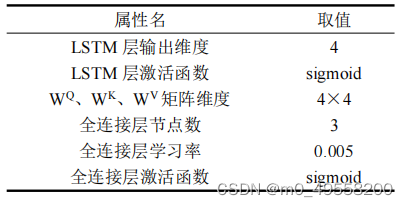三个权重矩阵的选取如下：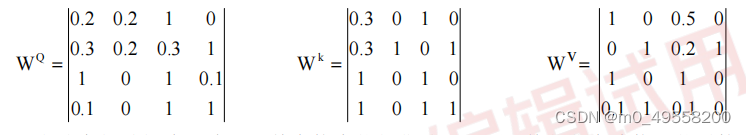最终的平均预测误差为10%左右，如果参数继续优化，误差会更小。

展开全文lstm 机器学习 python 大数据
• 1. LSTM模型 输入参数理解 （Long Short-Term Memory） lstm是RNN模型的一种变种模式，增加了输入门，遗忘门，输出门。 LSTM也是在时间序列预测中的常用模型。 小白我也是从这个模型入门来开始机器学习的坑。 LSTM的...
• 混合模型针对其他传统预测财务模型进行了测试，例如完整历史模型、恒定相关模型、单指数模型和多组模型。 在我们的实证研究中，ARIMA-LSTM 模型的预测能力明显优于所有其他金融模型。 我们的工作意味着值得考虑使用 ...
• 我基于matlab编写了用LSTM模型实现多步预测时间序列的程序代码。序列数据是我随机生成的，如果有自己的数据，就可以自己简单改一下代码，读取txt或excel都可以。注意读取后的序列必须命名为行向量。代码最后还提供了...
• 基于LSTM模型的股票价格预测 基于LSTM模型的股票价格预测 基于LSTM模型的股票价格预测 基于LSTM模型的股票价格预测 基于LSTM模型的股票价格预测 基于LSTM模型的股票价格预测 基于LSTM模型的股票价格预测 基于...
• 我基于matlab2021版编写了用LSTM模型实现多步预测时间序列的程序代码，可以自己调整使用的数据“阶数”。序列数据是我随机生成的，如果有自己的数据，就可以自己简单改一下代码，读取txt或excel都可以。注意读取后的...
• LSTM实践（代码详解）（一） 首先学习LSTM的目的：对时序数据有一个更深层次的挖掘，对比深度学习方法和机器学习方法在特征提取上的优劣。 文章目录LSTM实践（代码详解）（一）一、LSTM博文推荐二、代码详解先贴...tensorflow
• 论文介绍的LIC-LSTM模型的源代码：分层交织卷积LSTM 源代码以匿名方式提交给Github，以符合加拿大人工智能会议的双盲审查程序 要在字符预测任务上训练两层LIC-LSTM模型，请运行main_cnn_lstm_multi.m 该软件中的Adam...
• 主要介绍了Python中利用LSTM模型进行时间序列预测分析的实现，文中通过示例代码介绍的非常详细，对大家的学习或者工作具有一定的参考学习价值，需要的朋友们下面随着小编来一起学习学习吧
• 背景：最近在用CRNN做COR，CRNN的R就是LSTM，从开始深度学习的多年来一直在回避的东西这次躲不过去了，就试着去完整理解一下。 1、我之前的误解。当输入LSTM的Tensor shape为[None, c, n]时，我之前认为会有 c个...自然语言处理
• LCTFP：基于 CNN 和 LSTM 的高速公路交通流量预测模型(python代码) 车站内运行脚本的各个站点的一个半小时内的交通流数据。txt 运行数据_preprocess py。包括读取的所有文件txt。原来的处理数据：数据采集​​、归一...
• 写在前面：这是翻译自colah的一篇博客，原文关于LSTM神经网络模型的理解写的非常直观、简单易懂，所以翻译过来帮助大家学习理解LSTM模型。 当然我不是按照原文一字不落的翻译，而是摘出其中对模型理解最有帮助的...LSTM
• 机场是一个空地交通系统,机场地面交通的预测不同于一般城市道路具有其特殊性。本研究以北京首都国际机场为研究对象,基于2016年8月1日-2017年...与线性模型ARIMA和VAR模型相比,深度学习算法LSTM模型具有更好的预测精度。
• 【原创】python利用长短期记忆模型lstm进行时间序列预测分析数据分析报告论文(附代码数据) .pdf【原创】python利用长短期记忆模型lstm进行时间序列预测分析数据分析报告论文(附代码数据) .pdf【原创】python利用长...
• 该资源库包含我们编写的会议论文“使用递归神经网络的LSTM模型预测足球比赛获胜者”的代码。 本文介绍了在循环神经网络中使用LSTM（长期短期记忆）单元的优势，并用它来预测足球比赛的结果。 数据集 此处使用的数据...
• ## pytorch实现BiLSTM代码

千次阅读 2022-03-22 15:29:37pytorch LSTM
• 长短期记忆（Long Short Term Memory，LSTM）网络是一种特殊的RNN模型，其特殊的结构设计使得它可以避免长期依赖问题，记住很早时刻的信息是LSTM的默认行为，而不需要专门为此付出很大代价。 普通的RNN模型中，其...神经网络 自然语言处理
• 我基于Matlab2021编写了一个BO-LSTM算法，其中BO指的是贝叶斯（Bayesian）算法，利用BO算法对LSTM模型的超参数进行优化选择，包括采取的历史回归长度、隐藏层数、隐藏层单元数、单元随机丢弃率、初始学习率，该程序...
• 我就废话不多说了，大家还是直接看代码吧~ print(np.shape(X))#(1920, 45, 20) X=sequence.pad_sequences(X, maxlen=100, padding='post') print(np.shape(X))#(1920, 100, 20) model = Sequential() model.add...
• ## LSTM源码解析

千次阅读 2021-04-19 19:17:00
相比如卷积的过程，LSTM更加复杂，虽然看了一些博客后抽象上理解了，但还是想通过送入实际的值，调试代码来观察整个流程中的每一步来加深对其的理解。 本文以tensorflow-1.10版本为例，在eager模式下来进行lstm源码...
• 我基于Matlab2021编写了一个BO-LSTM算法，其中BO指的是贝叶斯（Bayesian）算法，利用BO算法对LSTM模型的超参数进行优化选择，包括采取的历史回归长度、隐藏层数、隐藏层单元数、单元随机丢弃率、初始学习率，该程序...matlab lstm
• 本文主要依据 Pytorch 中LSTM官方文档，对其中的模型参数、输入、输出进行详细解释。 目录 基本原理 模型参数 Parameters 输入Inputs: input, (h_0, c_0) 输出Outputs: output, (h_n, c_n) 变量Variables ...pytorch lstm...